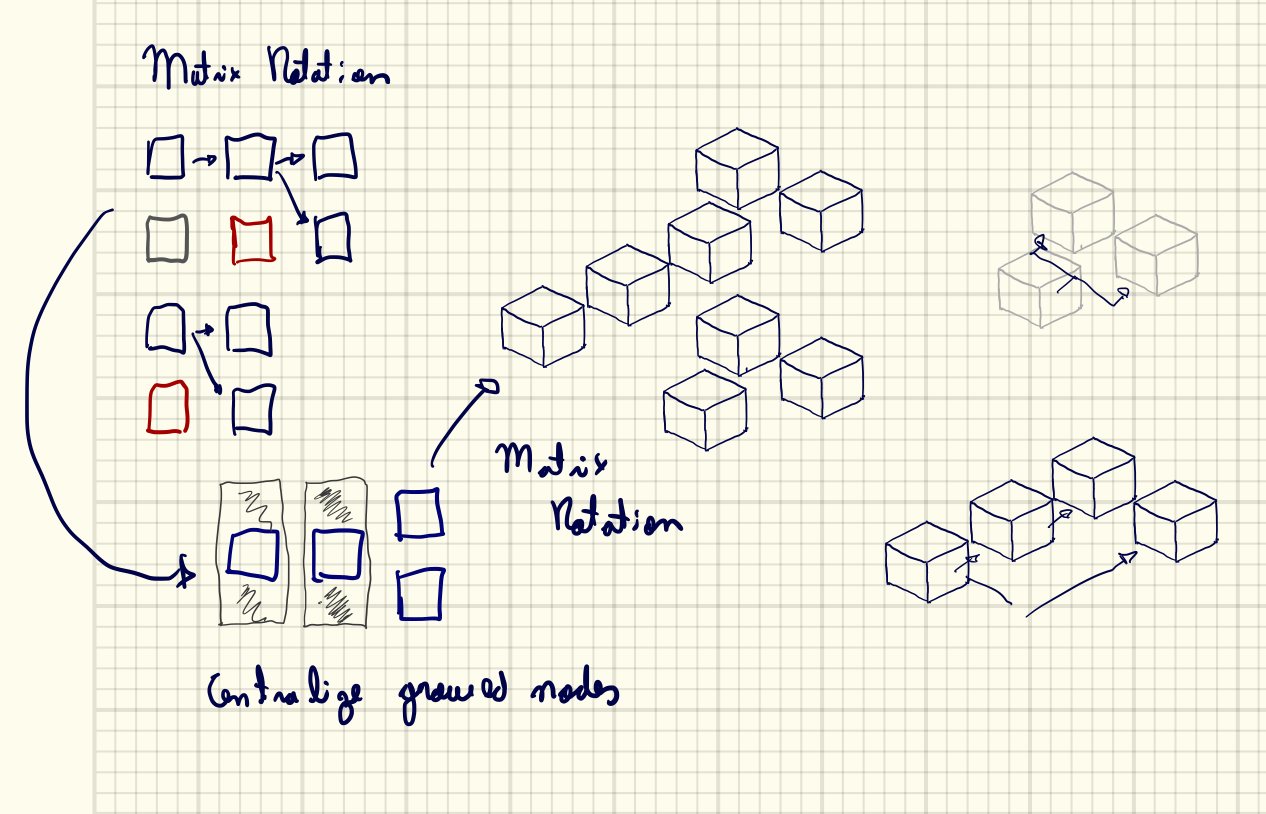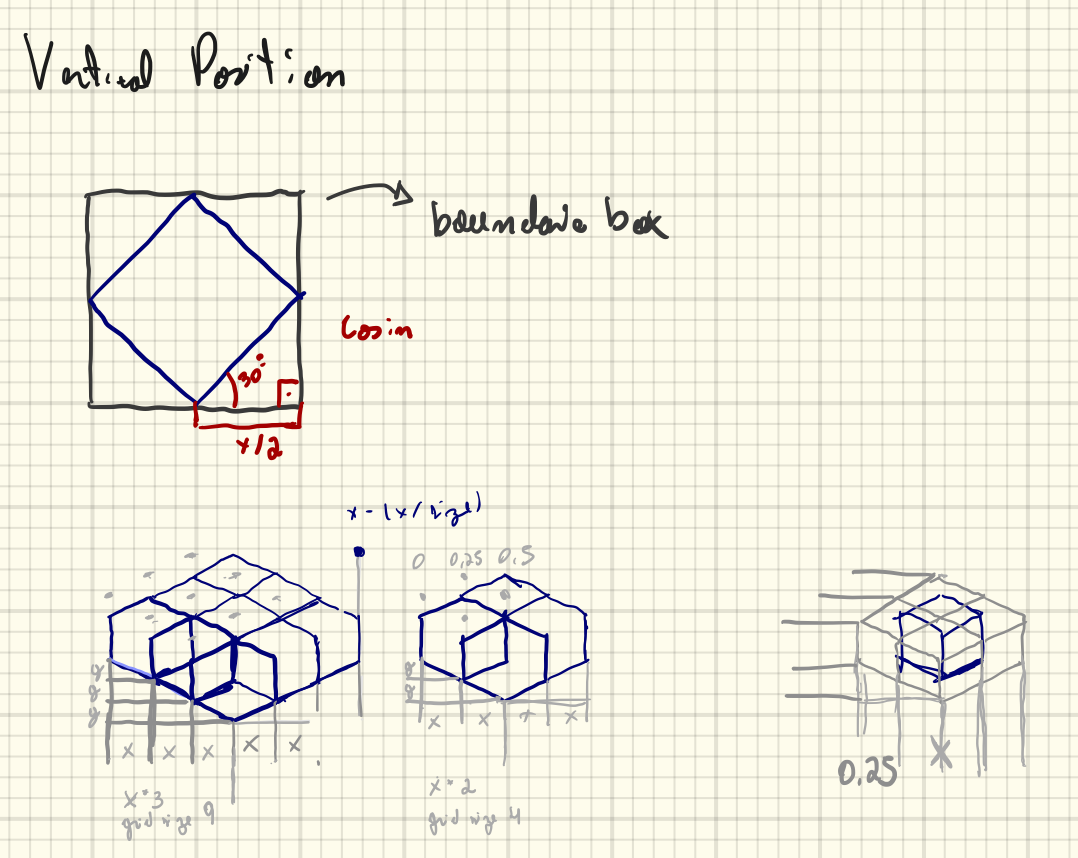# Algorithm Graphs Analytics¶

This section will expose how analytics graphs works.

Analytics system follow the steps below.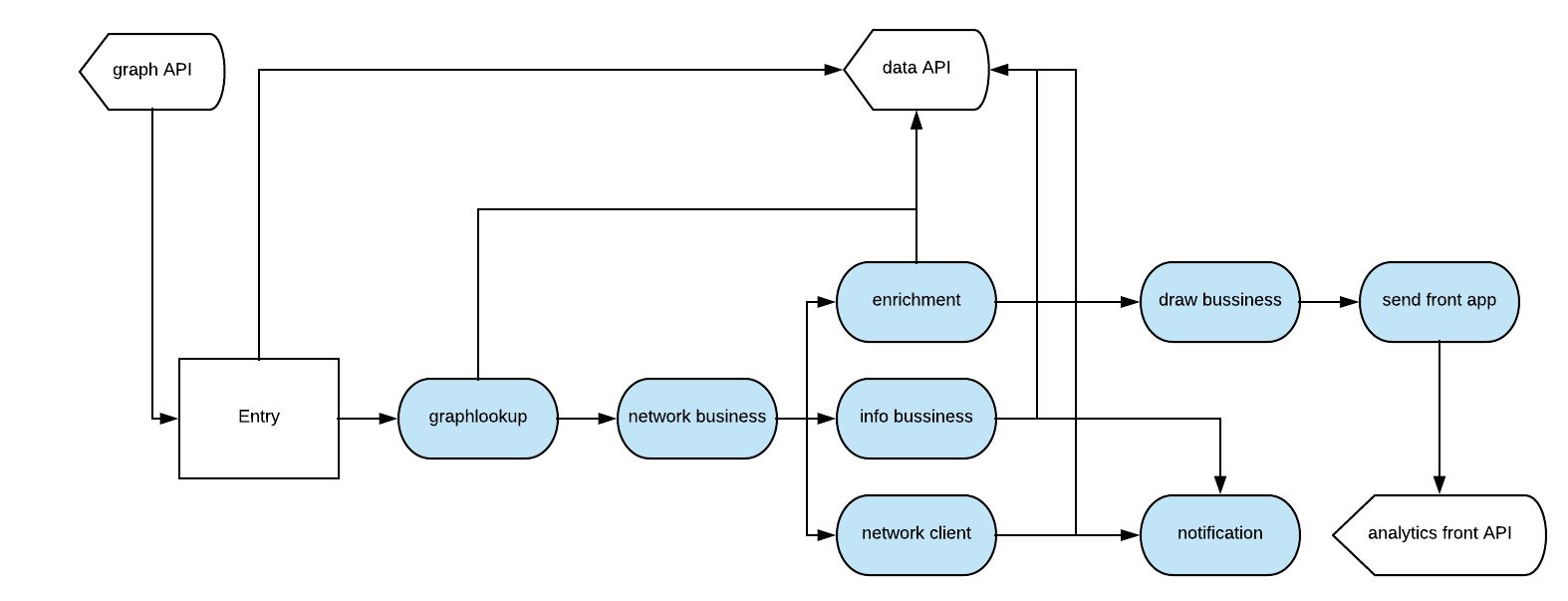## Making mongodb graph lookup¶

Graph lookup will create a python dict using mongodb graph, and all application entities have a dep field, its a list with app objects [id, name].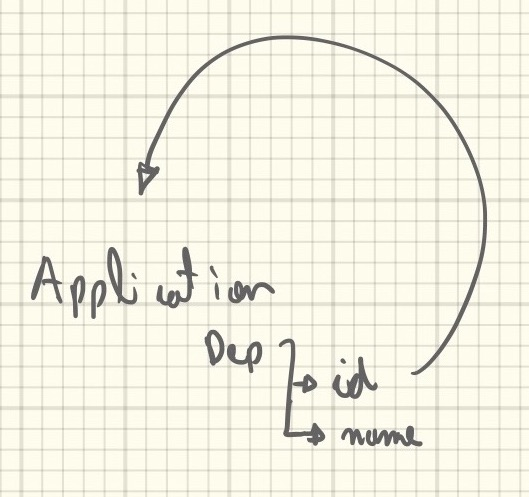## Creating a networkX graph¶

After graph sends to network business, basically transform python object to networkX.

We have a recursive function inside each node on the tree, this order will apply a lot of rules creating a new graph tree with better UI order, like in these moments we handle with conflicts, double dependencies, orders and etc.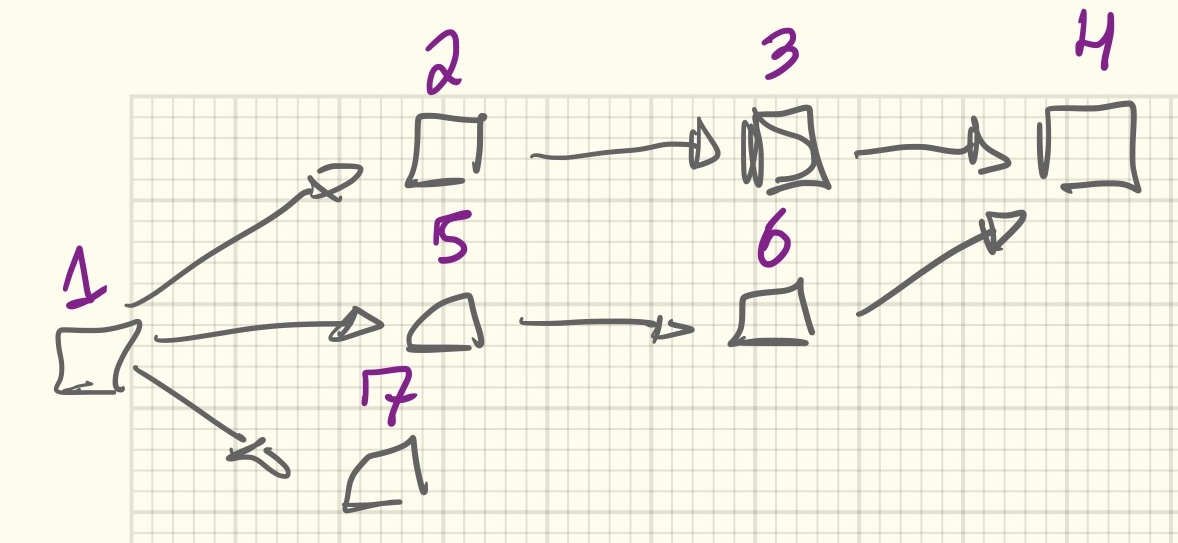Code example showing the recursive function

```def _recursive_draw(self, app, i=0, OHelper=HelperOrderedSuccers):
if i > 30:
return

for item in app:
if not self._grid.in_index(item):
node = self._graph.nodes[item]

succ = OHelper(helper).get_succers()
self._recursive_draw(succ, i + 1)
```

In these graphs we can see all rules applied on graph to create a best dependency tree. Each recursive tick try to figure out certainty pattern, if found apply a transformation. For example The child balance pattern try to find a multiple child in one parent, if happen then create a dummy item on parent layer.

## Rules¶

Growing node

• When: If the node have more than one child, growing the node to be equal of the number of child
• Transform: Set the node size to be equal of number of child

Child Balance

• When: If the parent node have more than two child.
• Transform: Create a dummy item beside to node parent.

Chess Pawn

• When: If the app it’s an entry point and have parent.
• Transform: Skipped one column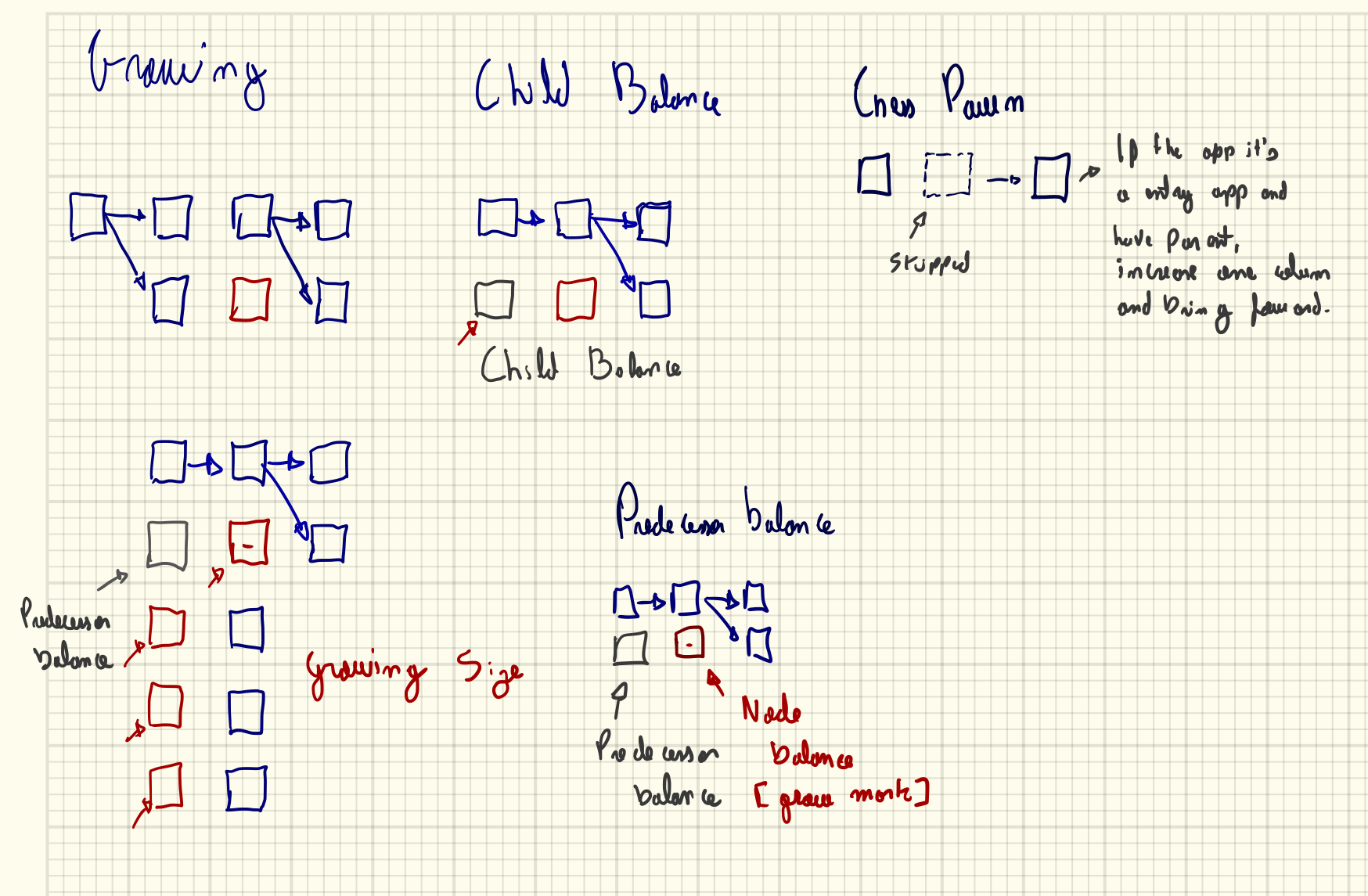Chess horse

• When: If the node have a top obstacle which other nodes point out to a common dependency.
• Transform: First push back the dependency to a clear column, and after create a dummy path to the new column.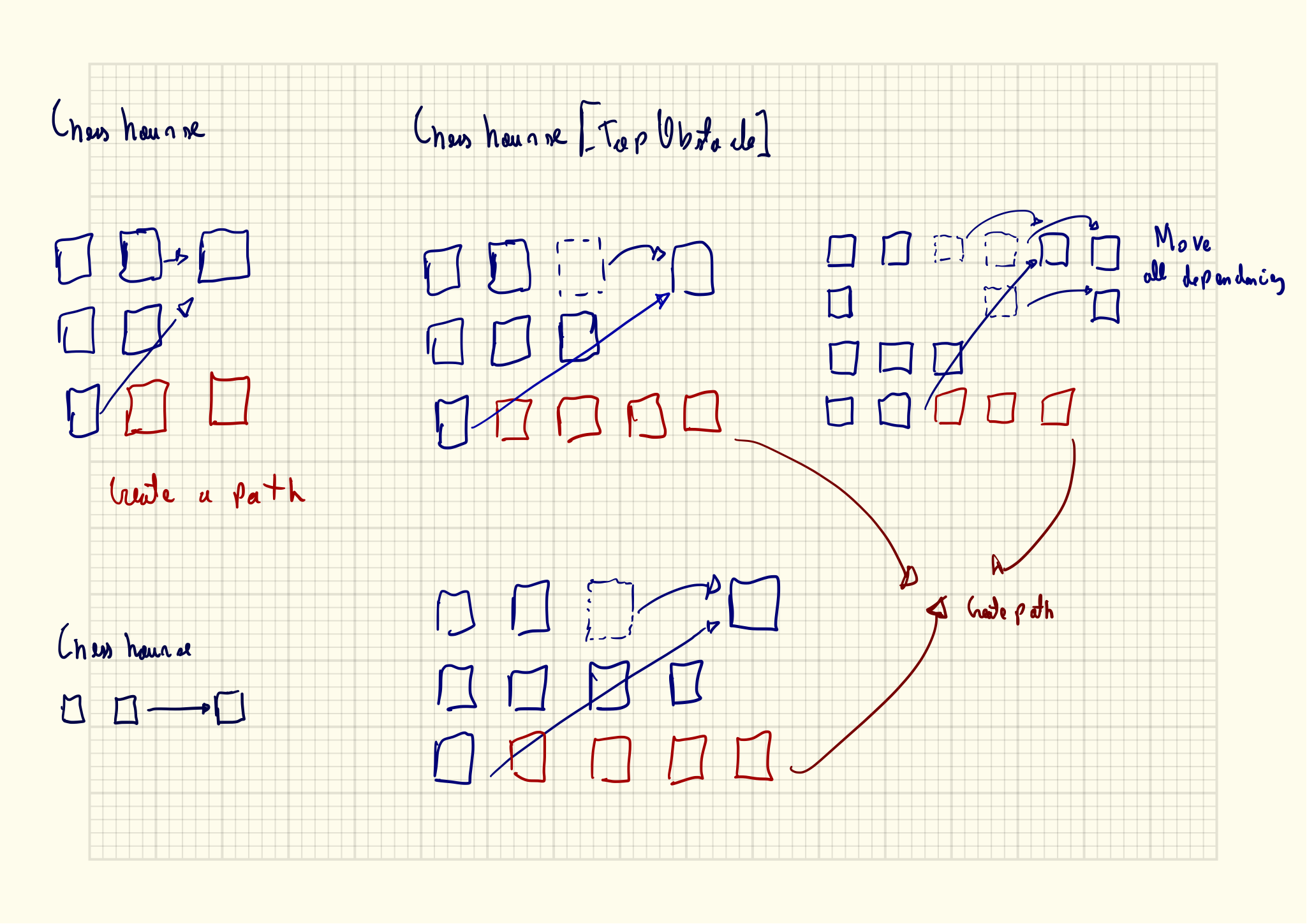Clear rows

• When: After finish the recursive loop, figure out empty columns.
• Transform: Delete these column and rebalance the grid.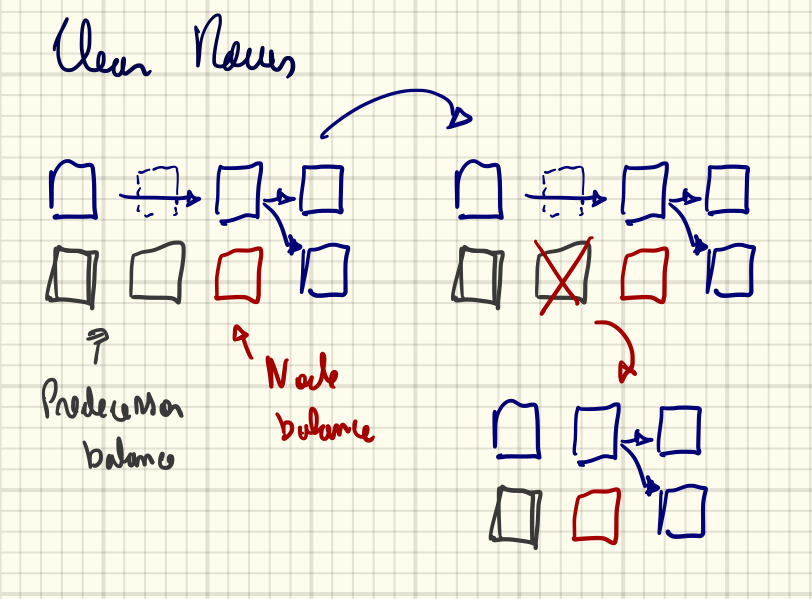## Enrichment data¶

Next step is an enrichment layer with getting all server data used by each graph.

The enrichment step received two dataset a json python dict represents a graph tree, a matrix position grid. One worker will get all applications accordingly with graph tree creating a new dataset called servers.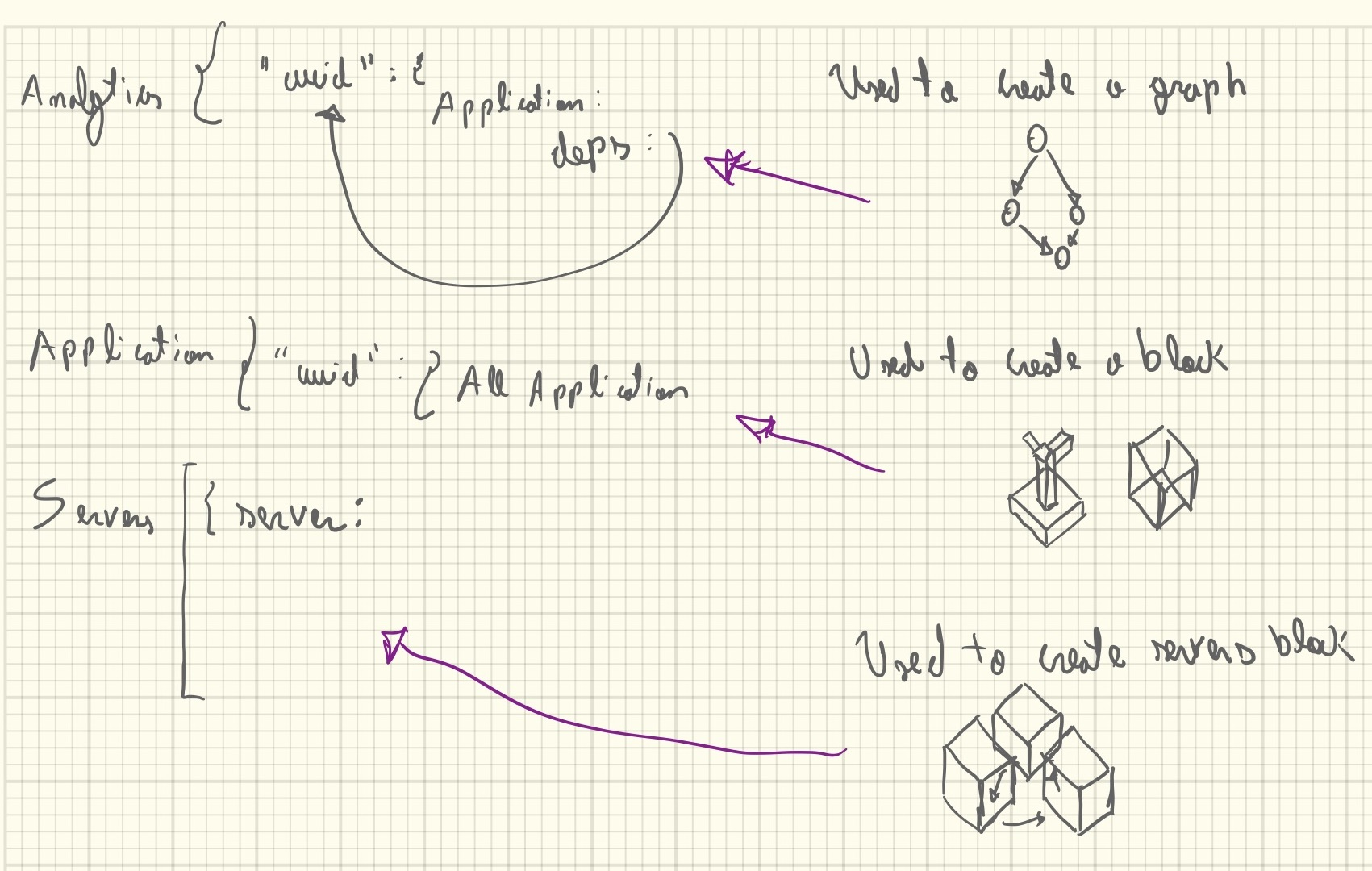## Draw layers¶

Now its draw time, where we have three datasets, one its a graph tree, second its matrix od each position and the last its list of servers.